## Thursday, January 31, 2019

### January 2019 Algebra 1 Regents, Part II

The following are some of the multiple questions from the January 2019 New York State Common Core Algebra I Regents exam.
The answers to Part I can be found here
The answers to Parts III and IV can be found here

### January 2019 Algebra I, Part II

Each correct answer is worth up to 2 credits. Partial credit can be given. Work must be shown or explained.

25. Solve algebraically for x: 3600 + 1.02x < 2000 + 1.04x

Subtract 2000 from both sides. Then subtract 1.02x from each side.
Finally, divide both sides by 0.02.

3600 + 1.02x < 2000 + 1.04x
1600 < 0.02x
80000 < x

26. The number of people who attended school's last six basketball games increased as the teamed neared the state sectional games. The table below shows the data.

 Game 13 14 15 16 17 18 Attendance 348 435 522 609 696 783

State the type of function that best fits the given data. Justify your choice of a function type.

A linear function is best because there is a constant rate of change of 87.
435 - 348 = 87
522 - 435 = 87
609 - 522 = 87, etc.
At this point, even if the rest weren't exactly 87, a linear function would still be the best fit.

27. Solve x2 - 8x - 9 = 0 algebraically
Explain the first step you used to solve the given equation.

Answer: If you graph it on the calculator, you will not get full credit. However, you can graph it if you wish to check your answer.
If you look for the factors of -9 that add up to -8, you will get -9 and +1

So x2 - 8x - 9 = 0 factors into
(x - 9)(x + 1) = 0
Then x - 9 = 0 or x + 1 = 0
So x = 9 or x = -1

You could have also, for example, completed the square or used the quadratic formula. In either case, you needed to explain what you were doing to get the second point.

28. The graph of f(t) models the height, in feet, that a bee is flying above the grown with respect to the time it traveled in t seconds.

State all time intervals when the bee's rate of change is zero feet per second. Explain your reasoning.

When the bee's rate of change is zero, the slope of the line will be zero. This means the parts of the graph that are horizontal line segments.
This intervals are between 2 and 6 seconds and again at 14-15 seconds.

29. Graph the function f(x) = 2x - 7 on the set of axes below.
If g(x) = 1.5x - 3, determine if f(x) > g(x) when x = 4. Justify your answer.

The graph will look like the exponential parent function y = 2x but translated seven units down.

g(4) = 1.5(4) - 3 = 6 - 3 = 3.
f(4) = 24 - 7 = 9. (You didn't have to show this if you included the point (4, 9) on the graph.
So f(4) > g(4).
You also could have graph g(x) to compare the points. If you did this, be sure to label both lines!

30. Determine algebraically the zeroes of f(x) = 3x3 + 21x2 + 36x

As with the earlier problem, if you graph the equation, you can check your answer, but you will not get full credit for doing it that way.
Any time you have a number greater than one as a coefficient for the first term, or if the exponent is greater than 2, look for common factors you might be able to remove.
3 is a factor of all three terms, and so is x.

f(x) = 3x3 + 21x2 + 36x
f(x) = 3x(x2 + 7x + 12)
f(x) = 3x(x + 3)(x + 4)

If 3x(x + 3)(x + 4) = 0 then
x = 0 or x = -3 or x = -4.
The zeroes of f(x) are 0, -3 and -4.

31. Santina is considering a vacation and has obtained high-temperature data from the last two weeks for Miami and Los Angeles.

Which location has the least variability in temperatures? Explain how you arrived at your answer.

The range of temperatures in Miami is 87 - 60 = 27
The range of temperatures in Los Angeles is 74 - 61 = 13

A similar question came up on the August Regents, and there were multiple acceptable answers.
For example, you could have used the Interquartile Range (Q3 - Q1), which you could have gotten if you had put all the data into two separate lists on your calculator and performed "1-Var Stats".
Or you could have compared standard deviations, similarly by making lists in your calculator.
These methods require extra work. Some students may have chosen these because comparing the range might appear to be "too easy".

32. Solve the quadratic equation below for the exact values of x.

4x2 - 5 = 75

Since there is no x1 term, it would be easier to use square roots than trying to factor the equation (in this blogger's opinion).
Add 5 to both sides: 4x2 = 80
Divide by 4: x2 = 20
Square root: x = + SQRT(20)

Note that "exact value" means leave it in radical form. DO NOT write a rounded decimal, not even one that has 10 decimal places. It won't be the exact value.

End of Part II

How did you do?

### Get Hype!

(Click on the comic if you can't see the full image.)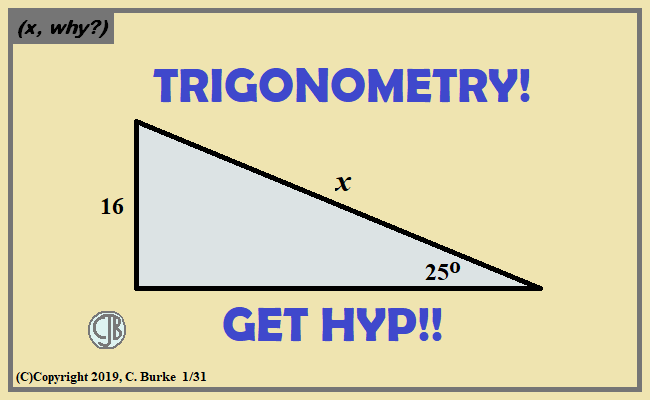But can you avoid SIN?

Come back often for more funny math and geeky comics.## Tuesday, January 29, 2019

### Data-Driven

(Click on the comic if you can't see the full image.)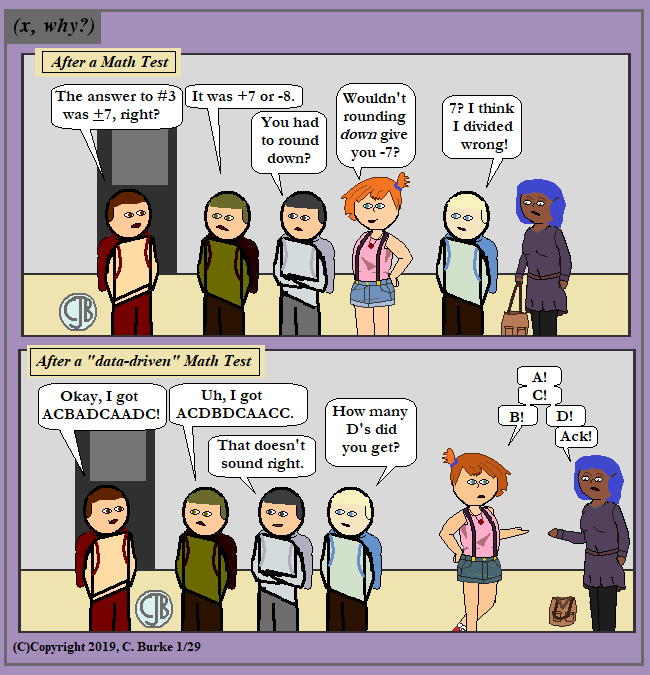''Discussion'' that I've overheard after different types of tests.

Yes, I get that "data" is important, but giving everyone the same exact multiple-choice test in a math class? For every exam? Ridiculous. And then people who should know better wonder why students have problems with open-ended problems on standardized testing. Or why they just can't explain a concept.

And don't get me started on how easy it is to cheat on a single-version multiple-choice test. At least, let me make two versions and I'll key in the results myself! (The problems with multiple versions of a computerized exam start with the kids needing to know in advance who has which version of the test and having to mark their bubble sheet accordingly. And if they mark it incorrectly or not at all, it's a waste of effort.)

Come back often for more funny math and geeky comics.## Wednesday, January 23, 2019

### August 2018 Common Core Algebra 1 Regents, Part I (mult choice)

The following are some of the multiple questions from the recent August 2018 New York State Common Core Algebra I Regents exam.
The answers to Part II can be found here
The answers to Parts III and IV can be found here

Omitted images will be added soon.

### August 2018 Algebra I, Part I

Each correct answer is worth up to 2 credits. No partial credit. Work need not be shown.

1. The number of bacteria grown in a lab can be modeled by P(t) = 300 • 24t, where t is the number of hours. Which expression is equivalent to P(t)?

24 = 16, so 24t = so (24)4t = 16t

2. During physical education class, Andrew recorded the exercise times in minutes and heart rates in beats per minute (bpm) of four of his classmates. Which table best represents a linear model of exercise time and heart rate?

Table 1 shows a linear model. There is a constant rate of change of 5 bpm per 1 minute.

3. David correctly factored the expression m2 - 12m - 64. Which expression did he write?

Answer: (3) (m - 16)(m + 4)
If you graph the original expression, you would see the zeroes are at -4 and 16. Flip the signs for the factored form.
If you multiply the choices, you will see that Choice (1) has a middle tern of -16m and (2) has a middle term of 0.
The factors of -64 that add up to -12 are -16 and +4.

4. The solution to -2(1 - 4x) = 3x + 8 is

Again, you could graph both sides of the equation and find the point of intersection.
And you can work backward from the choices (if you don't have a problem with fractions).
-2(1 - 4x) = 3x + 8
-2 + 8x = 3x + 8
8x = 3x + 10
5x = 10
x = 2

Check: -2(1 - 4(2)) = 3(2) + 8?
-2(-7) = 6 + 8
14 = 14

5. The graph of f(x) is shown below.
What is the value of f(3)?

Look at the graph. When x = -3, f(x) = 6.

6. If the function f(x) = x2 has the domain {0, 1, 4, 9}, what is its range?

Answer: (2) {0, 1, 16, 81}
The domain is the set of x values (the input to the function), and the range is the set of y values (the output of the function.)
The output is the square of the input. And squared numbers are always positive, so (4) makes no sense.
(1) shows the positive square root function. (3) shows both positive and negative and would NOT be a function.

7. The expression 4x2 - 25 is equivalent to

Answer: (3) (2x + 5)(2x - 5)
Difference of Squares rule. 4x2 is a perfect square. 25 is a perfect square.
Break it into two conjugates with the square root of each term. (Conjugates are the same two terms but one has a plus and one has a minus. When you multiply them, the inner and outer terms will "cancel".)

8. Compared to the graph of f(x) = x2, the graph of g(x) = (x - 2)2 + 3 is the result of translating f(x)

Answer: (3) 2 units right and 3 units up
First of all, this should have been a "gimme" because "2 units left and 3 units up" wasn't a choice.
The +3 shifts the graph UP, which eliminates (1) and (4).
The -2 shifts the graph to the RIGHT, which is (3). "Down" in (2) is just silly.

9. Lizzy has 30 coins that total \$4.80. All of her coins are dimes, D, and quarters, Q. Which system of equations models this situation?

Answer: (2) D + Q = 30 ; .10D + .25Q = 4.80
The sum of the coins is 30. The value of those coins .10D + .25Q

10. Gretchen has \$50 that she can spend at the fair. Ride tickets cost \$1.25 each and game tickets cost \$2 each. She wants to go on a minimum of 10 rides and play at least 12 games.
Which system of inequalities represents this situation when r is the number of ride tickets purchased and g is the number of game tickets purchased?

Answer: (2) 1.25r + 2g < 50, r > 10, g > 12
The most see can spend if \$50, so less than or equal to. Eliminate (1) and (4).
The minimum numbers are 10 rides and 12 games, so greater than or equal to.
This is a "silly" question based on the choices. The third sentence pretty much tells you that the two inequalities involving r and g have to have the same symbol. They are only the same in one of the four choices.

11. Three functions are shown [IMAGE TO BE ADDED]
Which statement is true?

Answer: (4) The y-intercept for g(x) is greater than the y-intercept for both f(x) and h(x).
The y-intercept for f(x) is 1 (from the graph).
The y-intercept for g(x) is g(0) = 30 + 2 = 1 + 2 = 3.
The y-intercept for h(x) is -1 (from the table).
Even if you thought 30 was 0, and g(0) was 2, you still should have gotten this correct.
Note that choice (3) could not be correct without choice (1) also being correct, so (3) could be eliminated.

12. Olivia entered a baking contest. As part of the contest, she needs to demonstrate how to measure a gallon of milk if she only has a teaspoon measure. She converts the measurement using the ratios below:

4 quarts/1 gallon * 2 pints/1 quart * 2 cups/1 pint * 1/4 cup/4 tablespoons * 3 teaspoons/1 tablespoon

Which ratio is incorrectly written in Olivia's conversion?

Short answer: in each case, the larger unit is on the bottom, except for this ratio.
Longer answer: to find the number of teaspoons in a gallon, all the in-between units need to cancel out, meaning that they will appear once in the numerator and once in the denominator. After pints are converted to cups, cups need to be converted to tablespoons. For this to happen, you need to know the number of tablespoons per cup, not cups per tablespoon.

13. If y = 3x3 + x2 - 5 and z = x2 - 12, which polynomial is equivalent to 2(y + z)?

Answer: (1) 6x3 + 4x2 - 34
3x3 + x2 - 5 + x2 - 12 = 3x3 + 2x2 - 17.
Multiply that sum by 2 and you get: 6x3 + 4x2 - 34

14. An outdoor club conducted a survey of its members. The members were asked to state their preference between skiing and snowboarding. Each member had to pick one. Of the 60 males, 45 stated they preferred to snowboard. Twenty-two of the 60 females preferred to ski. What is the relative frequency that a male prefers to ski?

45 out of 60 males preferred to snowboard, so 15 out of 60 preferred to ski. (They had to choose one of the two.)
15/60 = 0.25.

15. When the function g(x) = {5x, x < 3; x2 + 4, x > 3} is graphed correctly, how should the points be drawn on the graph for an x-value of 3?

Answer: (4) a closed circle at (3,15) and an open circle at (3,13)
When x = 3, the first condition is used, so there is a closed circle at 5(3) = 15.
The second condition has a boundary for x = 3, so there is an open circle for (3)2 + 4, which is 9 + 4 = 13.

16. If f(x) = 2x2 + x - 3, which equation can be used to determine the zeros of the function?

Answer: (2) 0 = (2x + 3)(x - 1)
Factor the function. Choices (3) and (4) cannot be used because they contain subtraction, not just factors.
Alternatively, you can graph the original function, along with each choice, to see which gives you the same graph.
I. x represents the number of dogs at the shelter per day.
II. x represents the number of volunteers at the shelter per day.
III. 30 represents the shelter’s total expenses per day.
IV. 30 represents the shelter’s nonfood expenses per day.

17. Each day, a local dog shelter spends an average of \$2.40 on food per dog. The manager estimates the shelter’s daily expenses, assuming there is at least one dog in the shelter, using the function E(x) = 30 + 2.40x.
Which statements regarding the function E(x) are correct?

The independent variable is the number of dogs. The number of volunteers doesn't matter in this function, and there is no information about them. E(x) is the daily expenses, not 30, which is the nonfood expenses, other than the \$2.40 spent per dog.
So statements I and IV are correct.

18. Which point is not in the solution set of the equation 3y + 2 = x2 - 5x + 17?

Zeroes of a function. Factored form.
The shortcut for the graphing calculator is to put the following into y1
y1 = (x2 - 5x + 17 - 2) / 3
You don't need to be neat about it.

Otherwise, plug in the values and see what makes a true statement:
(-2, 10): 3(10) + 2 = (-2)2 - 5(-2) + 17
32 + 2 = 4 + 10 + 17
34 =/= 31, Choice (1) is not a solution.

Check the others:
(-1, 7): 3(7) + 2 = (-1)2 - 5(-1) + 17
21 + 2 = 1 + 5 + 17
23 = 23, solution

(2, 3): 3(3) + 2 = (2)2 - 5(2) + 17
9 + 2 = 4 - 10 + 17
11 = 11, solution

(5, 5): 3(5) + 2 = (5)2 - 5(5) + 17
15 + 2 = 25 - 25 + 17
17 = 17, solution

19. The functions f(x) and g(x) are graphed below. [IMAGE OMITTED]
Based on the graph, the solutions to the equation f(x) = g(x) are

Answer: (3) the x-values of the points of intersection
In any system of equations, you are looking for the points of intersection. In particular, with two functions, you are looking for the values of x that make the two functions (the y values) equal.

20. For the sequence -27, -12, 3, 18, …, the expression that defines the nth term where a1 = 27 is

Answer: (4) -27 + 15(n - 1)
The initial term is -27. The common difference (18 - 3 or 3 - (-12)) is 15, which is obvious from the choices. The common difference gets the variable, so choices (1) and (2) are eliminated.
When n = 1, an = a1 = -27
However, if you add 15(1) to -27, you get -12, not -27, so choice (3) is no good.
In choice (4), by subtracting 1 from 1, you multiply the 15 by 0, and the term goes away, leaving only the -27, which is what we want.

21. The data obtained from a random sample of track athletes showed that as the foot size of the athlete decreased, the average running speed decreased. Which statement is best supported by the data?

Answer: (3) The sample of track athletes shows a correlation between foot size and running speed.
There is a correlation between the two -- we can see that there is some relationship.
However, we don't know that one thing causes the other, so you can't say that there is a causal relationship.

Final note: Look at the word again. It does not say "casual" relationship, which is something two people might be engaged in. (Yes, I bring this up because people read it incorrectly the first time.)

22. Which system of equations will yield the same solution as the system below?

x - y = 3
2x - 3y = -1

Answer: (3) 2x - 2y = 6; 2x - 3y = -1
In Choice (3), the first equation was multiplied by 2 while the second was left alone.
In Choice (1), the first equation was multiplied incorrectly by -2. The second term should have been + 2y.
In Choice (2), only the left side of the first equation was multiplied by -2; the right side was not.
In Choice (4), the first equation was multiply incorrectly by 3. The second term should be - 3y.

23. Which of the three situations given below is best modeled by an exponential function?
I. A bacteria culture doubles in size every day.
II. A plant grows by 1 inch every 4 days.
III. The population of a town declines by 5% every 3 years.

In Situation I, the bacteria doubles, then doubles again, etc. This is exponential.
In Situation II, the plant grows by the same amount, a constant rate. This is linear.
In Situation III, the population decreases by 5% then that decreases by 5%, etc. This is exponential.

24. The length, width, and height of a rectangular box are represented by 2x, 3x + 1, and 5x - 6, respectively. When the volume is expressed as a polynomial in standard form, what is the coefficient of the 2nd term?

Volume = length X width X height
(2x)(3x + 1) = 6x2 + 2x
(6x2 + 2x)(5x - 6) = 30x3 - 36x2 + 10x2 - 12x
= 30x3 - 26x2 - 12x
The coefficient of the middle term is 26.

End of Part I

How did you do?

## Tuesday, January 22, 2019

### W. O. D. B. #2

(Click on the comic if you can't see the full image.)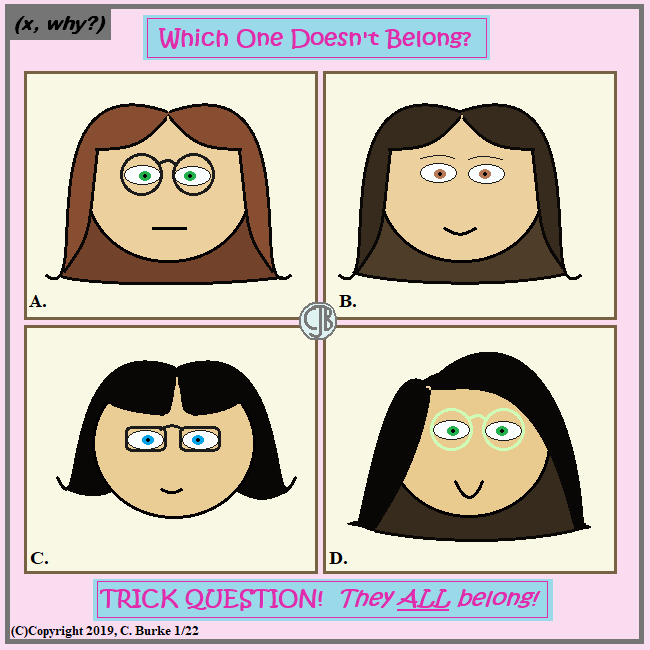Like I was going to say someone doesn't belong!

Inspired by four young ladies, who don't fall into these categories this neatly. I was making mental notes while learning their names when I noticed similarities amongst this group of friends.

As for the puzzle, I wanted there to be a "preferred" answer, but I added things that only apply to two, not three, of the images deliberately. It's like when someone says "B is the only right triangle" when two others are acute and the last is the only obtuse triangle. It doesn't quite fit.

Of course, I nearly messed myself up with this when, despite having it all worked out in advance, I suddenly decided to give C bangs. It looked great. Except that it messed up the answer to D. Such is life when creating puzzles.

Finally, I wanted to make sure that there were answers should someone print this out on a black and white printer.

If you answered, "A" because it's the only vowel, or the only letter with straight lines, GO AWAY!!!

Come back often for more funny math and geeky comics.## Friday, January 18, 2019

### (x, why?) Mini: USA!

(Click on the comic if you can't see the full image.)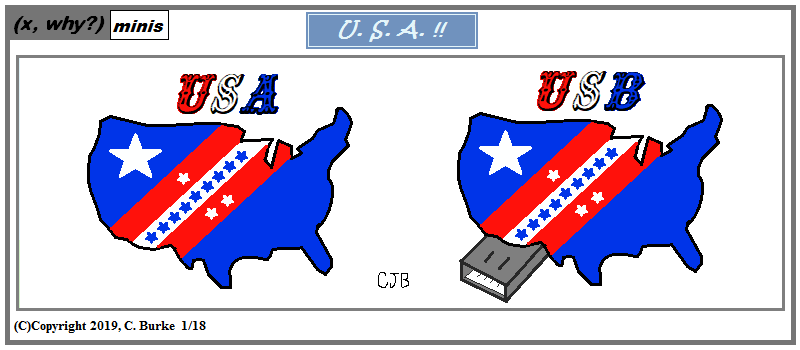I suppose you could fashion Alaska and Hawaii into some kind of cap. It'd be as accurate as many maps.

Assuming that this doesn't already exist, I'd be happy to sell my idea to someone who wants to make them!
Somehow, I assume that you can find this though.

Come back often for more funny math and geeky comics.## Wednesday, January 16, 2019

### August 2018 Algebra I Regents, Part III & IV

The following are some of the multiple questions from the August 2018 New York State Common Core Algebra I Regents exam.
The answers to Part I can be found here
The answers to Part II can be found here

### August 2018 Algebra I, Part III

Each correct answer is worth up to 4 credits. Partial credit can be given. Work must be shown or explained.

33. Sarah wants to buy a snowboard that has a total cost of \$850, including tax. She has already save \$135 for it. At the end of each week, she is paid \$96 for babysitting and is going to save three-quarters of that for the snowboard.
Write an inequality that can be used to determine the minimum number of weeks Sarah needs to babysit to have enough money to purchase the snowboard.
Determine and state the minimum number of full weeks Sarah needs to babysit to have enough money to purchase this snowboard.

Very simply: The first want you to just write the inequality, which you then use to answer the second part of the question.

She has 135, she is saving 3/4 of 96 per week for n weeks and needs more than 580.
135 + (3/4)(96)n > 580.

135 + 72n > 580
72n > 445
n > (445/72)
n > (445/72)
n > 6.18
Sarah needs to babysit for 7 full weeks to pay for the snowboard.
Remember: You cannot round *down* because then she will not have enough money. She will be a few dollars short.

34. A car was purchased for \$25,000. Research shows that the car has an average yearly depreciation rate of 18.5%.
Create a function that will determine the value, V(t), of the car t years after purchase.
Determine, to the nearest cent, how much the car will depreciate from year 3 to year 4.

1.00 - 0.185 = 0.815
V(t) = 25000(0.815)t

Use this formula to find the value of V(3) and V(4) and subtract them to find the difference. (You can also graph this in your calculator and check the Table of Values)
V(3) - V(4) = 25000(0.815)4 - 25000(0.815)3
= 13533.584 - 11029.871 = 2503.713 = \$2,503.71 depreciation between years 3 and 4.

35. Graph the following systems of inequalities on the set of axes below:

2y > 3x - 16
y + 2x > -5

Based upon your graph, explain why (6, 1) is a solution to this system and why (-6, 7) is not a solution to this system.

If you plan on using the graphing calculator, you will want to rewrite these inequalities in slope-intercept form. (At the very least, you will want to isolate the y on the left side.)

2y > 3x - 16
y > 3/2 x - 8
y + 2x > -5
y > -2x - 5

Notice that in the first case, there will be a solid line, and in the second, it will be broken. In both cases, you will be shading about the line, and the section shaded twice will be the solution. Remember to label both lines.

(6, 1) is in the solution because it is on the solid line, and points on the solid line are part of the solution.
(-6, 7) is not a solution because it is on the broken line, which is a boundary and not part of the solution set.

Note: if you draw you graph incorrectly, your explanation would need to match your graph. If somehow (-6, 7) was a part of your solution, you could try to explain that, but the better move would be to realize that you made a mistake and fix it!

36. Paul plans to have a rectangular garden adjacent to his garage. He will use 36 feet of fence to enclose three sides of the garden. The area of the garden, in square feet, can be modeled by f(w) = w(36 - 2w), where w is the width in feet.
On the set of axes below, sketch the graph of f(w).
Explain the meaning of the vertex in the context of the problem.

In the context of the problem, the vertex is the width of the garden that will produce the greatest area. When the width is 9 feet, the area will be 162 square feet.

### August 2018 Algebra I, Part IV

A correct answer is worth up to 6 credits. Partial credit can be given. Work must be shown or explained.

37. At the present time, Mrs. Bee’s age is six years more than four times her son’s age. Three years ago, she was seven times as old as her son was then.
If b represents Mrs. Bee’s age now and s represents her son’s age now, write a system of equations that could be used to model this scenario.
Use this system of equations to determine, algebraically, the ages of both Mrs. Bee and her son now.
Determine how many years from now Mrs. Bee will be three times as old as her son will be then.

Mrs. Bee (b) is (=) six years more than (+ 6 to the next item) four times her son's age (4s).
b = 4s + 6
Three years ago (b - 3), she was (=) seven times (7 *) as old as her son was then( (s-3) ).
b - 3 = 7(s - 3) -- Note the parentheses around (s-3)! Those are important!

b = 4s + 6
b - 3 = 7(s - 3) means b = 7(s - 3) + 3
So 4s + 6 = 7(s - 3) + 3)
4s + 6 = 7s - 21 + 3
4s + 6 = 7s - 18
24 = 3s
8 = s
b = 4(8) + 6 = 32 + 6 = 38.
Mrs. Bee is 38 now and her son is 8.
Check: 38 - 3 = 35. 7(8 - 3) = 7(5) = 35. Check!

In n years, when Mrs. Bee is three times older than her son, then her age then divided by the son's age then will equal three.
(38 + n) / (8 + n) = 3, or
(38 + n) = 3(8 + n)
38 + n = 24 + 3n
14 = 2n
7 = n. In seven years, Mrs. Bee will be three times her son's age.
Check: 38 + 7 = 45. 3(8 + 7) = 3(15) = 45. Check!

End of Part Exam

How did you do?

## Tuesday, January 15, 2019

### Famous Movie Math Quotes #5

(Click on the comic if you can't see the full image.)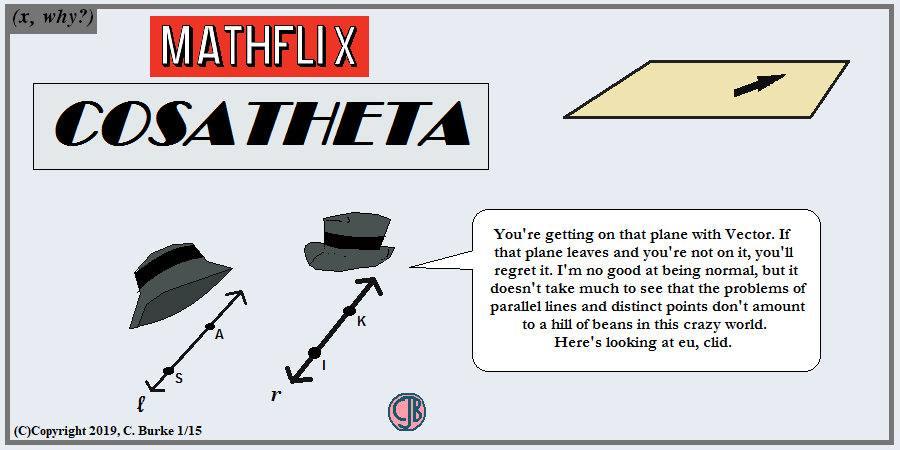"We'll always have Pairs" is #43.

I pared down the dialogue a bit. It wouldn't all fit, and it wouldn't all be funny or particularly mathy.

For the newcomers, the number 5 in the title is a reference to the AFI's Top 100 Movie Quotes list from ten years ago or so. I've done a couple of these over the years, but not in any particular order. Will I do more of these? Maybe not today, maybe not tomorrow, but soon ... or not so soon.

Come back often for more funny math and geeky comics.## Friday, January 11, 2019

### Clip - Art

(Click on the comic if you can't see the full image.)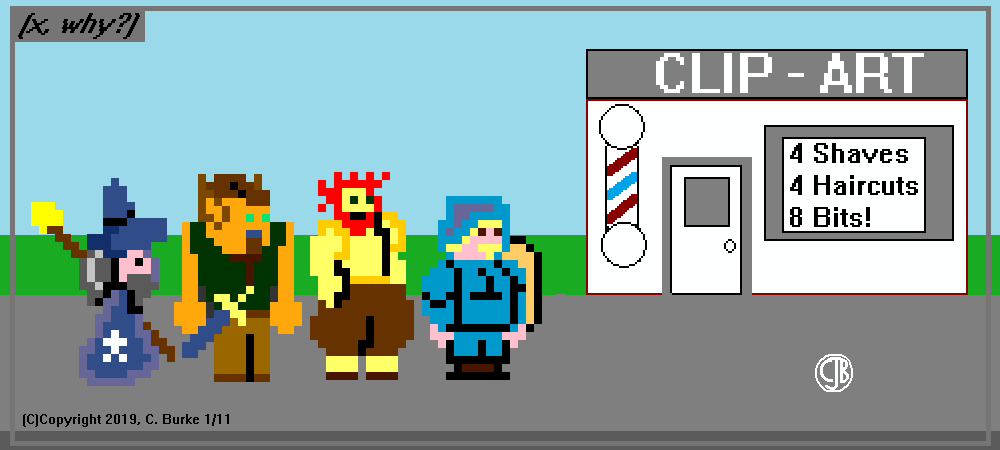That Art! He is the Clipper to the Stars!

Ten years in the making, at least. And I originally wanted to put some familiar head shots in there, like you see on barber walls.

I've had the joke, but not the means to do it. Then, on a whim, I checked the web for 8-bit creators, and found a couple. I created a witch in no time. And then I nearly crashed my iPad when I tried using some of the site's functions to either save or email the picture. Seriously, if was a half-hour or more before my iPad was responding, and I was afraid I would have to do another factory reset.

Luckily, the second site was a little better and PC friendly. I recreated my witch, print-screened and saved it that way. Then I re-colored it to match my original witch, which I liked better. (Which witch? The first one.)

I made the next three in a couple of days, and passed them by the expert: my son. I thought they came out good, but didn't know what was sticking on the side of the second guy's head. I said I was going for "Elf". Oh, well. I changed the coloring a little.

Next problem was that the joke was ALWAYS "Four SHAVES, Four Haircuts". Only one guy had a beard -- and one of the others was female.

First try was to create the pirate, who I thought was "okay" but I wasn't happy with his eyepatch or his hat. Keep in mind, he came before either of the two pirate jokes that I already posted this week. He could've tied them all together very nicely. (He might've been part of the reason I came up with the other two jokes, and well as the play on "Barber".)

But I liked the witch, and I thought that a wizard wouldn't be a difficult transition. So I went that route instead.

For those who are interested, the original artwork is posted below. The knight always had a beard, even before I "remembered" the joke. Witch, elf, barbarian (part satyr?), knight, pirate, wizard.

I hope you like them. I could try to recreate them sometime, but it won't be easy though.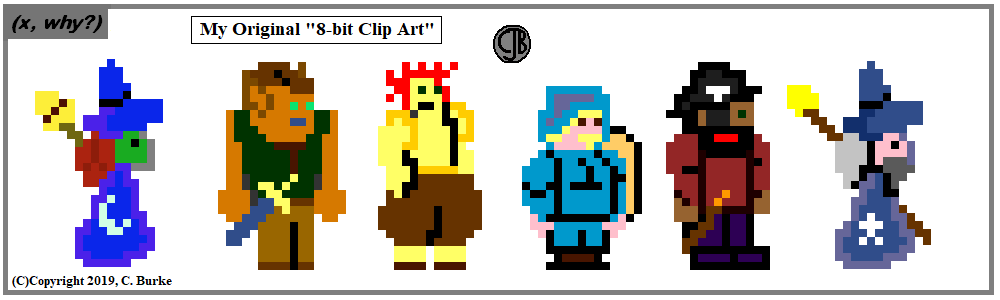Come back often for more funny math and geeky comics.## Wednesday, January 09, 2019

### Barbary Pirates, Part II

(Click on the comic if you can't see the full image.)Many a battle were close shaves!

I apologize for nothing.

Come back often for more funny math and geeky comics.## Tuesday, January 08, 2019

### August 2018 Algebra I Regents, Part II

The following are some of the multiple questions from the August 2018 New York State Common Core Algebra I Regents exam.
The answers to Part I can be found here
The answers to Parts III and IV can be found here

### August 2018 Algebra I, Part II

Each correct answer is worth up to 2 credits. Partial credit can be given. Work must be shown or explained.

25. Explain how to determine the zeros of f(x) = (x + 3)(x -1)(x - 8).
State the zeros of the function.

To find the zeroes of a function in factored form, set each of the factors equal to 0 and solve for x.

The zeroes of this function are:

x + 3 = 0 OR x - 1 = 0 OR x - 8 = 0
x = -3 OR x = 1 OR x = 8

26. Four relations are shown below.

State which relation(s) are functions.
Explain why the other relation(s) are not functions.

III and IV are functions.
I is not a function because it fails the vertical line test. When x = 6, there are two values of y, 4 and 6. This is not allowed.
II is not a function because the x values 1 and 2 are repeated with different y values.

Added information: In III, the x values in the table do not repeat. In IV, the quadratic equation given is a parabola, which is a function. There is only one possible outcome when you square a number.

27. The table below represents the height of a bird above the ground during flight, with P(t) representing height in feet and t representing time in seconds.

Calculate the average rate of change from 3 to 9 seconds, in feet per second.

P(3) = 6.26 and P(9) = 3.41.
The average rate of change is (3.41 - 6.26) / (9 - 3) = -2.85 / 6 = -0.475 feet per second.

0 = 2x2 + 3x - 10

Check the discriminant: b2 - 4ac
32 - 4(2)(-10) = 9 - (-80) = 89
The square root of 89 is an irrational number, so the solutions to the equation are irrational.

29. The formula for converting degrees Fahrenheit (F) to degrees Kelvin (K) is:

K = (5/9) (F + 459.67)
Solve for F, in terms of K.

K = (5/9) (F + 459.67)
(9/5) K = F + 459.67
(9/5) K - 459.67 = F

30. Solve the following equation by completing the square:

x2 + 4x = 2

Take half of 4 (which is 2) and square it (which is 4). Add that to both sides.

x2 + 4x = 2
x2 + 4x + 4 = 2 + 4
Now factor
(x + 2)2 = 6
x + 2 = + SQRT (6)
x = -2 + SQRT (6)

Since the wanted an exact value, leave the answer in fraction form because it's a repeating decimal. DO NOT ROUND.

31. The students in Mrs. Lankford’s 4th and 6th period Algebra classes took the same test. The results of the scores are shown in the following table:

Based on these data, which class has the largest spread of test scores? Explain how you arrived at your answer.

The 4th Period has the larger spread.
The sigma value (the second column), which is used to find variance and standard deviation, is greater for 4th period, so the spread will be greater.
Also, if you calculate the Interquartile Range, Q3 - Q1, you will get the following results:
4th Period: 87.5 - 69 = 18.5
6th Period: 88 - 71.5 = 16.5
4th Period has the greater IQR so it has the greater spread of the data.

Personal note: I'm not fond of this question. A little to vague. However, they chose data that would give the same answer whichever method was chosen. I'm not sure if calculating only the range would have been good enough, but if you search online, you will see that the range is listed as one measure of the spread of data. (Certainly, the others are better measures, but it doesn't mean the other method isn't one.)

32. Write the first five terms of the recursive sequence defined below.

a1 = 0
an = 2(an - 1)2 - 1, for n > 1

a1 = 0
a2 = 2(0)2 - 1 = 0 - 1 = -1
a3 = 2(-1)2 - 1 = 2(1) - 1 = 1
a4 = 2(1)2 - 1 = 2(1) - 1 = 1
a5 = 2(1)2 - 1 = 2(1) - 1 = 1

0, -1, 1, 1, 1

Note: every term after the 3rd will be 1.

End of Part II

How did you do?

## Monday, January 07, 2019

### (x, why?) Mini: Barbary Pirates, Part I

(Click on the comic if you can't see the full image.)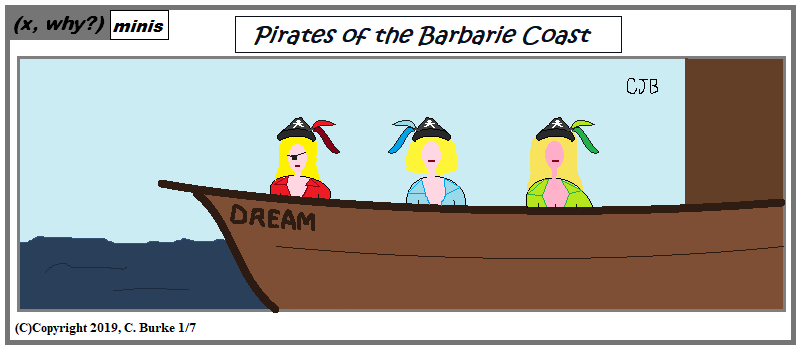Ken you defeat the Barbarie Dream boat? You can really test you Mettle.

Funny, I work on dialogue and/or narration for a while, as well as considering props ... and then just kept it simple.

Come back often for more funny math and geeky comics.## Thursday, January 03, 2019

### Happy Thirdsday

(Click on the comic if you can't see the full image.)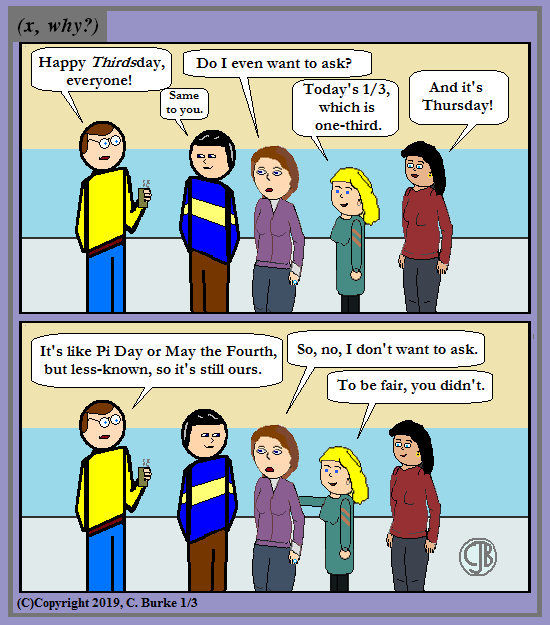Enjoy it before it gets over-commercialized! Only happens on Thursday, on average, once every seven years!

We don't get another one until 2030!

Come back often for more funny math and geeky comics.## Tuesday, January 01, 2019

### Happy New Year 2019!

(Click on the comic if you can't see the full image.)Happy New Year! I'm getting tired of these.

Seriously, if I kept a regular schedule and produced close to 150 comics per year, then "filler" comics like this one would be okay. But I've only done 1400 or so in 11 years, which is a smaller average.

I don't want to be a holiday comic any more than I want to be an "in memorium" comic. Basically, I need a fresh take on New Years Eve and New Years Day. The "Eve" is the party day, while the "Day" is the number change. I've done binary and other weird combinations already.

And I'm less impressed about finding some mathematical equation that equals 2019 (or whichever year) because in the waning days of December, there will be tweets galore on social media with so many sums of squares and such. (And quite a few will be just expanded binary operations.)

In summary, if something brilliant doesn't occur to me -- and frankly, I don't think I'll ever top 2013 or the original bad pun for 2008 -- next year will likely be a random character stating "Happy New Year. This is 2020." Okay, I guess I need a Hugh Downs and Barbara Walters by then.

Come back often for more funny math and geeky comics.